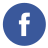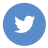### JavaScript sort strings

javascript

To sort an array of strings in JavaScript, you can use the sort() method of the Array object.

Here is an example of how you can use sort() to sort an array of strings in ascending order:

``````const words = ['cherry', 'apple', 'blueberry'];
words.sort();
console.log(words);  // Output: ["apple", "blueberry", "cherry"]``````

You can also use the sort() method with a compare function as the argument to control the sort order. The compare function should return a negative value if the first argument should be sorted before the second argument, a positive value if the first argument should be sorted after the second argument, and 0 if the two arguments are equal.

Here is an example of how you can use a compare function with sort() to sort the strings in descending order:

``````const words = ['cherry', 'apple', 'blueberry'];
words.sort((a, b) => {
if (a < b) {
return 1;
}
if (a > b) {
return -1;
}
return 0;
});
console.log(words);  // Output: ["cherry", "blueberry", "apple"]``````

You can also use the localeCompare() method to compare strings in a case-sensitive or case-insensitive way, using the rules of a specified locale.

Here is an example of how you can use localeCompare() with sort() to sort the strings in case-insensitive ascending order:

``````const words = ['cherry', 'Apple', 'blueberry'];
words.sort((a, b) => a.localeCompare(b, undefined, { sensitivity: 'base' }));
console.log(words);  // Output: ["Apple", "blueberry", "cherry"]``````